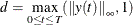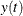# The MODEL Procedure

### Numerical Integration

The differential equation system is numerically integrated to obtain a solution for the derivative variables at each data point. The integration is performed by evaluating the provided model at multiple points between each data point. The integration method used is a variable order, variable step-size backward difference scheme; for more detailed information, see Aiken (1985); Byrne and Hindmarsh (1975). The step size or time step is chosen to satisfy a local truncation error requirement. The term truncation error comes from the fact that the integration scheme uses a truncated series expansion of the integrated function to do the integration. Because the series is truncated, the integration scheme is within the truncation error of the true value.

To further improve the accuracy of the integration, the total integration time is broken up into small intervals (time steps or step sizes), and the integration scheme is applied to those intervals. The integration at each time step uses the values computed at the previous time step so that the truncation error tends to accumulate. It is usually not possible to estimate the global error with much precision. The best that can be done is to monitor and to control the local truncation error, which is the truncation error committed at each time step relative towhereis the integrated variable. Furthermore, thes are dynamically scaled to within two orders of magnitude of one to keep the error monitoring well-behaved.

The local truncation error requirement defaults to 1.0E–9. You can specify the LTEBOUND= option to modify that requirement. The LTEBOUND= option is a relative measure of accuracy, so a value smaller than 1.0E–10 is usually not practical. A larger bound increases the speed of the simulation and estimation but decreases the accuracy of the results. If the LTEBOUND= option is set too small, the integrator is not able to take time steps small enough to satisfy the local truncation error requirement and still have enough machine precision to compute the results. Since the integrations are scaled to within 1.0E–2 of one, the simulated values should be correct to at least seven decimal places.

There is a default minimum time step of 1.0E–14. This minimum time step is controlled by the MINTIMESTEP= option and the machine epsilon. If the minimum time step is smaller than the machine epsilon times the final time value, the minimum time step is increased automatically.

For the points between each observation in the data set, the values for nonintegrated variables in the data set are obtained from a linear interpolation from the two closest points. Lagged variables can be used with integrations, but their values are discrete and are not interpolated between points. Lagging, therefore, can then be used to input step functions into the integration.

The derivatives necessary for estimation (the gradient with respect to the parameters) and goal seeking (the Jacobian) are computed by numerically integrating analytical derivatives. The accuracy of the derivatives is controlled by the same integration techniques mentioned previously.